# Energy (science)

(Redirected from Energy)

Energy is a property of a system that produces action (makes things happen) or, in some cases, has the "potential" to make things happen. For example, energy can put vehicles into motion, it can change the temperature of objects and it can transform matter from one form to another, e.g., energy will turn solid water (ice) of 0 °C into liquid water of 0 °C. Energy lights our cities, lets our planes fly, and runs machinery in factories. It warms and cools our bodies and homes, cooks our food, plays our recorded music, and gives us pictures on television.

Quantitatively, energy is a measurable physical quantity of a system and has the dimension M(L / T)2 (mass times length squared over time squared). The corresponding SI (metric) unit is the joule (which equals 1 kg·m2/s2); other measurement units are ergs, calories, watt-hours, Btu, etc. All these units have the dimension M(L / T)2, and if one finds a physical property of a system with these dimensions, one is entitled to call that quantity a part of the energy of the system.

It is difficult, or perhaps impossible, to give an all-embracing definition of energy, because energy exists in many forms, such as kinetic or mechanical energy, potential energy, thermal energy or heat, light, electrical energy, chemical energy, nuclear energy, etc. Indeed, it took scientists a long time to realize that the different manifestations of energy are really the same property, and that in all cases it may rightfully carry the same name (energy). From the middle of the 18th to the middle of 19th century, scientists came to realize that the different forms of energy can be converted into each other, and moreover that no energy is lost in the conversion processes.

Let us look at the conventional coal-fired power plant as a practical example of the conversion of energy. Such a plant takes as input coal (carbon) and air (oxygen). These two raw materials combine, i.e., coal is burned, and combustion energy, a form of heat, is generated. Combustion energy is converted into electrical energy which is transported to cities and factories through high-voltage power lines. It would be very nice, and would go a long way in solving the energy crisis, if all of the combustion energy would be converted into electrical energy. Unfortunately, this is not the case, the laws of physics do not allow it. Thermodynamics dictates that the larger part of the combustion energy is turned into non-useable thermal energy, which in practice is carried off by cooling water. Although the cooling water heated by the electricity plant is of little practical use because of its relatively low temperature, it still contains thermal energy that (theoretically not practically) could be used to perform work. At lower ambient temperatures a larger part of the thermal waste energy is converted into useful electrical energy and in the hypothetical case of zero K (−273 °C) ambient temperature all of the thermal energy in the warmed cooling water is converted into electrical energy, which shows that thermal energy is indeed a form of energy. In any case, the thermal energy of the cooling water is important in the energy balance of the electricity plant:

Combustion energy → electrical energy + thermal energy

Because energy is conserved, the combustion energy is equal to the sum of the electrical and the thermal energy..

The different manifestations of energy are discussed in more detail in the following sections of this article.

## Energy in classical mechanics

To keep the discussion simple we will consider a point particle of mass m in one-dimensional space. That is the position of m at time t is given by x(t). For more details and extension to the three-dimensional case, see classical mechanics. Let us assume that a force F(x) is acting on the particle. As an example one may think here of a mass in the gravitational field of the earth. The one dimensional space in this example is a line perpendicular to the surface of the earth. Actually, the case considered is slightly more complicated, namely F is taken to be a function of x, while the gravitational force F does not depend on x. (At least near the surface of the earth. The expression for F close to the surface is: F = mg, where g is the gravitational acceleration, a quantity of approximate value 9.8 m/s².) Further, by considering F(x) the case of frictional (dissipative, non-conservative) forces that are not functions of position (but often functions of only the velocity of the mass) is excluded.

### Potential energy

In classical mechanics one can define the potential energy of a system as the work the system can perform potentially. If work is done by the system its potential energy decreases. If work is done on the system its potential energy increases. As stated, the physical system that will be considered is the simplest one possible: a particle of mass m in a one-dimensional space with a force field F(x).

Imagine, as an example, the great scientist Galileo Galilei, carrying a mass, say a cannon ball, up the stairs of a church tower. Doing this, Galileo has to work against the gravitational force, which pulls the cannon ball downward. The work ΔW performed by Galileo on the cannon ball (the system) is proportional to the gain in height Δx and the absolute value |F| of the force. The work ΔW is positive and the force is directed downward (F < 0), so we have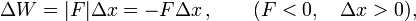$\Delta W = |F| \Delta x = -F \Delta x \,,\qquad (F < 0, \quad \Delta x > 0),$

for the work performed by Galileo on the system during his carrying it up the stairs over a height Δx. The corresponding gain ΔU in the potential energy of the cannon ball, is the work done on it by Galileo,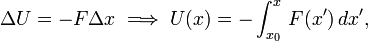$\Delta U = - F\Delta x \; \Longrightarrow\; U(x) = - \int_{x_0}^x \, F(x')\, dx',$

where we made the choice of zero of potential energy: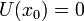$U(x_0) = 0 \,$. In this example the obvious choice of x0 is the base of the tower, i.e., x0 is the street level. By the fundamental theorem of integral calculus, we have the important expression that relates force F(x) and potential energy U(x),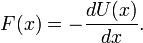$F(x) = - \frac{dU(x)}{dx}.$

#### Potential energy in three dimensions

The generalisation to three dimensions of the definition of potential energy U(r) is,$\mathbf{F}(\mathbf{r}) \equiv -\boldsymbol{\nabla} U(\mathbf{r}),$

where the gradient is the vector operator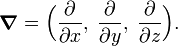$\boldsymbol{\nabla} = \Big( \frac{\partial}{\partial x},\; \frac{\partial}{\partial y},\; \frac{\partial}{\partial z}\Big).$

In order that this generalization can be made, or in other words, that a potential energy U(r) can be defined, it is necessary that the force field F(r) is conservative (non-dissipative). That is, F(r) must satisfy Euler's reciprocity equations,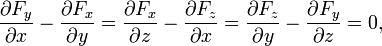$\frac{\partial F_y}{\partial x} - \frac{\partial F_x}{\partial y}= \frac{\partial F_x}{\partial z} - \frac{\partial F_z}{\partial x}= \frac{\partial F_z}{\partial y} - \frac{\partial F_y}{\partial z}= 0,$

which can be written more concisely by the use of the curl,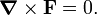$\boldsymbol{\nabla} \times \mathbf{F} = 0.$

### Kinetic energy

Besides potential energy, classical mechanics knows another form of energy: kinetic energy. Suppose Galileo drops the mass to the bottom of the tower after arriving at its top. The mass will pick up speed, (we will neglect air resistance, which will put some brake on the falling mass and generate some heat, friction with air being a dissipative force) and get the kinetic energy$T \equiv \tfrac{1}{2} m v^2 \quad \hbox{with} \quad v \equiv \frac{d x}{d t} ,$

where the speed of the particle is the absolute value of its velocity v.

### Equivalence of kinetic and potential energy

This dropping of mass off the top of the church tower is a good example of conversion of energy: potential energy is converted in kinetic energy. Herewith energy is conserved, that is, the sum of kinetic and potential energy is constant in time. Indeed,$\frac{d}{dt} (T+U) = \frac{1}{2}m \frac{dv^2}{dt} + \frac{d U}{dt} = m v \frac{dv}{dt} + \frac{dU}{dx} \frac{dx}{dt} = m v a - F v, \quad\hbox{with}\quad a \equiv \frac{dv}{dt} ,$

where a is the acceleration of the mass. Invoke Newton's second law (see classical mechanics):$F = m a \quad\hbox{and}\quad\frac{d}{dt} (T+U) = m v a - ma v = 0,$

and it is proved that the time derivative vanishes of the total energy ET + U. That is, E is a conserved, time-independent, property of the cannon ball falling from the tower.

### Collisions

Finally, one may wonder what happens when the particle, dropped by Galileo from the top of the tower, hits the ground. Here we have a collision of two bodies, the earth and the dropped particle. The collision can be elastic, in which case no energy is dissipated. If we take the mass of the earth to be infinite, the particle bounces up with the same kinetic energy that it had when it hit the earth. That is, its speed |v | remains the same, but the sign of v changes. The momentum mv of the particle changes by −2mv on collision, which seems contradictory to the law of conservation of momentum. The latter conservation law holds when there are no outside forces acting on the physical system consisting of the earth and the dropped particle. Since it was assumed implicitly that no outside forces are present, we indeed expect conservation of momentum. To explain this apparent violation, note that the earth receives the absolute value of momentum M |V | = 2m|v | from the collision, where M is the mass of the earth and V is the velocity of the earth gained by the collision. When M goes to infinity, V goes to zero. Hence, for infinite mass the earth absorbs momentum without changing velocity and without picking up kinetic energy. This is why the kinetic energy of the bouncing particle is conserved.

A collision may be inelastic: the particle may break up in pieces which fly off with kinetic energy and the earth will absorb the remaining kinetic energy of the falling particle. This absorption is by increase of the internal energy of the earth, which in general implies some warming up of the earth. Of course, the law of energy conservation still holds: the kinetic energy of the broken particle pieces and the increase of the internal energy of the earth add up to the kinetic energy of the dropped particle.

As a final remark: most collisions are somewhere in between elastic and completely inelastic. The particle will bounce back some height, losing some kinetic energy that is transferred to the earth as an increase of the earth's internal energy. Also the internal energy of the dropped particle may increase somewhat by the collision. This must also be included in the energy balance.

## Energy in thermodynamics

### Energy from heat

A thermodynamical system is a physical system with an extra property: temperature (T). When two thermodynamical systems of unequal temperature are in thermal contact, heat will flow spontaneously from the warmest (highest temperature) system to the coldest (lowest temperature) system. This heat flow will decrease the temperature of the warmer system and increase the temperature of the colder. The heat flow will be sustained until equilibrium is reached and the two systems have the same temperature. At equilibrium the spontaneous heat flow stops.

By using a heat pump it is possible to transfer energy from a colder to a warmer system. This requires input of mechanical or electrical work. The energy transferred from the colder to the warmer system is also called heat.

Earlier in this article, energy was defined in a hand waving manner as the capacity of a system to do work. Now the question arises whether exchange of heat, which is an exchange of energy, can perform work. Or, in other words, can the energy content of a heat bath be utilized to perform work? It is clear that in any case two systems of different temperatures are needed, otherwise heat will not flow. The first to recognize this clearly was William Thomson (Lord Kelvin).

A spontaneous heat flow is depicted in the figure on the right, where we see two heat baths, with T1 > T2. The circle in the middle designates a heat engine, a cyclic process in which heat is converted into work W. From the first law of thermodynamics follows that after a number of full cycles of the engine, when no net energy is stored in the engine,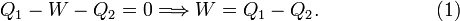$Q_1-W-Q_2 = 0 \Longrightarrow W = Q_1-Q_2. \qquad\qquad\qquad (1)$

The second law of thermodynamics states that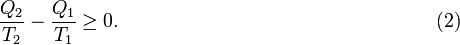$\frac{Q_2}{T_2} - \frac{Q_1}{T_1} \ge 0. \qquad\qquad\qquad\qquad\qquad\qquad\qquad\qquad\; (2)$

If we take the heat engine in the drawing to be the idealized Carnot engine that undergoes reversible changes, the equality sign holds. To obtain the theoretical upper bound to the efficiency of the process, we assume this to be the case. Multiplication of Eq. (2) by T2 / Q1 gives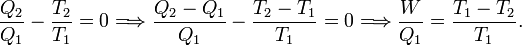$\frac{Q_2}{Q_1} - \frac{T_2}{T_1} = 0 \Longrightarrow \frac{Q_2 -Q_1}{Q_1} -\frac{T_2-T_1}{T_1} = 0 \Longrightarrow \frac{W}{Q_1} = \frac{T_1-T_2}{T_1}.$

Define the efficiency η by$\eta \;\stackrel{\mathrm{def}}{=}\; \frac{W}{Q_1}\quad\Longrightarrow\quad W = \eta\;Q_1$

and it follows that the efficiency is proportional to the temperature difference of the upper and lower temperature bath: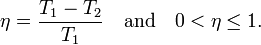$\eta = \frac{T_1-T_2}{T_1}\quad\hbox{and}\quad 0 < \eta \le 1.$

The work W is a fraction η of the heat Q1 delivered by the upper heat bath. For instance, if T1 = 500 0C and T2 = 20 0C , then η = 480/(273.15+500) = 0.62. That is, at most 62% of the heat delivered by the upper heat bath is converted into work, the remaining energy is lost to the lower heat bath.

One may wonder why the work W is related here to Q1. The answer is that the setup of the figure is a model for many engines. Historically, the model was first introduced by Sadi Carnot for the steam engine. The upper heat bath models the steam boiler which is held at a constant temperature T1 by burning fuel (in the days of the steam engine usually coal). During the cycle in the middle of the figure the steam drives a piston that performs work W. During this process, the steam cools down, and the steam is cooled down even further in the condenser, becoming liquid water again. The condenser takes away the rest heat Q2, which is not used any further, but given off to the environment (in this scheme the lower heat bath of ambient temperature T2 models the environment). The condensed water is led back from the condenser to the steam boiler and heated again, completing the cycle. So, the heat flow between two reservoirs of unequal temperature (the steam boiler and the environment) generates work plus a rest energy Q2. The fact that this rest energy appears has the consequence that only a fraction η of Q1, the heat obtained from burning fuel, can be used to do work. Since the burning of fuel is the determining factor in the cost of operating the engine, the efficiency is expressed as a fraction of Q1.

The same principle applies to combustion engines, for instance car engines, where the rest heat Q2 is given off to the environment through the car's radiator. The fact that only a fraction (about ¼) of the chemical energy stored in gasoline is converted into mechanical work (kinetic energy of the car) is not a design flaw, but a consequence of physical principles (the first and second law of thermodynamics). 

The three arrows in the figure can be reverted, in which case the figure depicts a heat pump, for instance a refrigerator or an air conditioner. Work is delivered to the system, usually by an electric motor, and heat Q2 is drawn from the lower temperature bath (for instance, the inside of a refrigerator). The heat Q1 is transported to the higher temperature heat bath (in the case of a refrigerator the air in the kitchen, in the case of an air conditioner the outside air). Here we see an illustration of the Clausius principle: it takes work W to extract the amount Q2 of heat from the low temperature bath. This is converted into the heat Q1 that is transported to the high temperature bath. Since a refrigerator gives off its heat to the kitchen, it cannot be used as an air conditioner. The work W done by its electric motor is converted into the net heat Q1Q2. Overall, the refrigerator acts as an electric heater, converting electric energy W > 0 into the net heat Q1Q2 > 0 that is given off to the surroundings of the refrigerator. By the same reasoning it is clear why an air conditioner needs an outlet outside the house for its rest heat.

In practical applications, such as power plants and combustion engines, it is hard to achieve a large efficiency factor (T1T2)/T1, because T2 is in practice always ambient temperature as it costs energy to obtain lower temperatures. The higher temperature T1 cannot be raised too much as it is restricted by the burning process, the material of burners, etc. Power plants have a typical efficiency of 38%.

### Work

Besides being able to exchange heat, a thermodynamic system can do also work on another system or on its environment, which decreases its internal energy U. Conversely, another system, or the environment, can do work on the system, increasing U. Above we already assumed that the exchange of energy by work was possible for the Carnot engine. Work can be mechanical, electrical, magnetic, chemical, and so on.

The standard textbook example of mechanical work regards a gas filled cylinder with a piston on top. Let the pressure inside the cylinder be p, the surface of the piston be S and the volume of the cylinder be V. If the piston is moved into cylinder over a distance Δx, an amount of work ΔW is performed on the gas which is equal to F Δx. By the definition of pressure the force F is equal to pS, so that the work is ΔW = pSΔx = pΔV, where we assume that p is constant under the small displacement of the piston. The internal energy increases by ΔU, while V decreases by ΔV, so that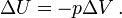$\Delta U = - p \Delta V\,.$

If the piston moves outward, the volume increases, the system performs work on its surroundings, costing it internal energy, and hence the sign in the equation covers this case as well.

The work performed on, or by, the system is of the form aΔb, where a does not depend on the size of the system (when we halve the volume of the system and its gas content the pressure p stays the same). The quantity a is an intensive parameter. The quantity b is linear in the size of the system, it is an extensive parameter. This is a general form for all expressions for work, they always involve an intensive/extensive parameter couple. Another example is the polarisation P (a macroscopic dipole) of a dielectric in a static electric field E. The work done by the field is EΔP. When we add an amount Δn mol of substance to a system, we increase its internal energy by μΔn, where μ is the chemical potential of the substance. This addition of substance can be seen as "chemical work" performed on the system. Even heat exchange fits this pattern, ΔQ = T ΔS, where the temperature T is an intensive and the entropy S is an extensive parameter.

## Chemical energy

A chemical reaction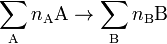$\sum_\mathrm{A} n_\mathrm{A} \mathrm{A} \rightarrow \sum_\mathrm{B} n_\mathrm{B} \mathrm{B}$

may be exothermic, in which case heat escapes from the reaction in the form of translational (external) energy of the molecules B and often radiation. Or, the reaction may be endothermic in which case heat must be supplied in order to let the reaction proceed. Exothermic reactions form a source of chemical energy.

Very often chemical reactions proceed at constant—usually ambient—pressure p. The reaction heat Q is then equal to the change in enthalpy ΔH of the reactants. Indeed, according to the first law of thermodynamics, we have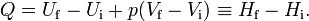$Q = U_\mathrm{f} - U_\mathrm{i} +p(V_\mathrm{f} -V_\mathrm{i}) \equiv H_\mathrm{f} - H_\mathrm{i}.$

Here Uf is the total internal energy of the final product molecules B and Ui of the initial molecules A. Since the reaction occurs at constant pressure p, the work term is p(VfVi). This term must be included in the energy balance of the first law. The thermodynamic state function "enthalpy" is by definition HU + pV. Note that an exothermic reaction is characterized by Hf < Hi, i.e., has a negative reaction enthalpy Δ HHf - Hi < 0. Correspondingly, an endothermic reaction has a positive reaction enthalpy.

In daily life the most important source of energy is the chemical energy obtained from the reaction called combustion. In this chemical reaction oxygen from the air reacts with a fuel, such as gasoline, coal, or natural gas, giving off heat. The fossil fuels contain carbon as the single most important element. Take graphite as an example:

C(graphite) + O2(g) → CO2(g)    ΔH = −393.6 kJ.

The second most important element contained in fossil fuels is hydrogen. The combustion reaction of gaseous hydrogen is

2H2(g) + O2(g) → 2H2O(l)    ΔH = −571.6 kJ
Gross combustion enthalpies
Fuel MJ/kg
Natural gas 55
Liquified petroleum gas 50
Aviation gasoline 46
Automotive gasoline 46
Kerosene 45
Diesel 46

Commercially available natural gas, LPG (liquified petroleum gas), gasoline, kerosine and diesel are mixtures of many hydrocarbons. The adjacent table presents some typical (approximate) values of their combustion enthalpies (often referred to as energy contents, heating values, caloric values or heats of combustion):

These values are per kilogram. Ordinary gasoline has a density of 0.78 kg/L, so that 1 L of gasoline has an energy content of approximately 36 MJ/L.

It is of some interest to give a crude estimate of energy consumption in daily life, indicating orders of magnitude only. Assume, therefore, that it takes 0.1 L (=0.079kg) of gasoline to drive a midsized car one km (corresponds to 23 miles to the gallon, 10 km to the liter). This car consumes an energy of roughly 3.6 MJ per km, which is close to 1 KWh/km (kilowatt-hour per kilometer). Hence, driving the car over one kilometer costs roughly the same energy as running a 1000 W electric appliance for an hour. Of course, this does not take into account the energy loss in the generation of the electricity. A power plant running on fossil fuel has typically an efficiency of 38%. If we include this number, we see that driving a midsized car over one kilometer costs the same energy as running a 380 W electric apparatus for an hour.

It may be, parenthetically, interesting to look at the energy consumption of an electric car. Most electric cars use roughly 0.20 kWh/km. Transportation of electricity and battery-charge losses are about 10%, so that an electric car has a net consumption of about 0.22 kWh/km. If we include in this number the 38% efficiency of power stations, we arrive at 0.58 kWh/km for an electric car, which may be compared to the 1 kWh for a gasoline car. Clearly, the gain in efficiency of an electric car over a gasoline car is due to the efficiency of a power station (38%) versus that of a car engine (25%). In both cases the less than 100% efficiency is due to unavoidable heat losses.

Another example: Suppose the members of a household drive on average 30000 km (19000 mile) per year in a midsized car. This costs 30 MWh/year. The average Western-world household uses 3.5 MWh of electricity per year. Including the energy loss at the power station, a household spends three times more energy on driving a car than on electricity.

## Electrostatic energy

Consider two point charges q1 and q2, a distance r12 apart. By Coulomb's law the one particle acts on the other with a force that is inversely proportional to the mutual distance squared,$F(r_{12}) = \frac{q_1q_2}{4\pi\epsilon_0 r_{12}^2},$

where ε0 is the vacuum permittivity. The forces on the two particles act along the line joining the particles. If the charges are of opposite charge, the forces are attractive, otherwise they are repulsive. As in classical mechanics, the work done by the force is minus force times distance. The work increases or decreases the potential energy of the system, so that the electrostatic energy of a system of two point charges is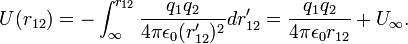$U(r_{12}) = - \int_{\infty}^{r_{12}} \frac{q_1q_2}{4\pi\epsilon_0 (r'_{12})^2} dr'_{12} = \frac{q_1q_2}{4\pi\epsilon_0 r_{12}} + U_{\infty}.$

The constant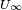$U_\infty$ can be chosen freely since its choice does not affect the electric field (minus the gradient of U), which is the physical quantity of concern. This freedom of choice is a form of gauge invariance. It is common to choose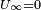$U_\infty = 0$.

Consider next a system of N point charges. The potential energy of the system is additive, hence the electrostatic energy of a system of N point charges is,$U = \sum_{i=1}^N \sum_{j > 1}^N \frac{q_i q_j}{4\pi\epsilon_0 r_{ij}} = \frac{1}{2} \sum_{i=1}^N \sum_{j=1 \atop j\ne i}^N \frac{q_iq_j}{4\pi\epsilon_0 r_{ij}}$

where the condition on the summation over j excludes the (infinite) self-energy. In the second equation the factor ½ is introduced to avoid counting the same interaction twice. This energy if of great importance in molecular physics, because a molecule can be seen as a collection of point charges.

This expression allows us to introduce a static potential (scalar) field due to a static charge distribution,$V(\mathbf{r}) \equiv \sum_{i=1}^N \frac{q_i}{4\pi\epsilon_0 | \mathbf{r}_i-\mathbf{r}| }.$

It is the work required to bring a single positive unit charge from infinity (where V is zero) to r. Or in other words, V(r) is the voltage difference between r and infinity, or, briefly, the electric potential at the point r due to the charge distribution.

## Electric energy

Consider a conducting wire of finite length with a static voltage difference V between the ends. The voltage difference will be kept constant, for instance, by a battery, or an electric generator. An electric current (a flow of positive charges) will run from positive to negative voltage. This electric current transports energy,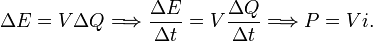$\Delta E = V \Delta Q \Longrightarrow \frac{\Delta E}{\Delta t} = V \frac{\Delta Q}{\Delta t} \Longrightarrow P = V i.$

Here P is power (energy/time, expressed in watt), i is (direct) current (charge/time, expressed in ampere) and V is voltage difference (expressed in volt).

The magnitude i of the current is determined by the apparatus (light bulbs, electric oven, electric motors, etc.) that the wire runs through. All these will take up power. The power can be in the form of heat generated per unit time: i2R, where R is the resistance of (part of) the wire. If, for instance, the current runs through an electric heater, part of the energy is converted to heat, i.e., electric power is converted into an energy flow from the heater outward, warming up the surroundings. If the current runs through an electric motor, electric power is converted to mechanical power, i.e., electrical energy is converted to mechanical work. In contrast to the conversion of heat to work, this process is practically loss-less. The only loss is by heating of the wires inside the motor, because of resistance of the wires (again i2R).

In general, the energy carried by an electric current is measured in kWh (kilowatt×hour), instead of the regular SI unit of energy joule (J). Note that 1 kWh = 3600 kJ, since 1 W = 1 A⋅V (ampere×volt) and 1 A = 1 coulomb/second (C/s) and 1 C⋅V (coulomb×volt) = 1 J.

## Equivalence of energy and mass

Einstein showed in his theory of special relativity that the energy of a free particle of (rest) mass m and speed v is equal to$E = \gamma m c^2\quad\hbox{with}\quad \gamma = \frac{1}{\sqrt{1-\frac{v^2}{c^2}}},$

where c = the speed of light in a vacuum = 299,792,458 m/s

Using the Taylor series$\frac{1}{\sqrt{1+x}} = 1 - \frac{1}{2}x + \frac{3}{8} x^2 +\cdots,$

we find that the energy of the free particle becomes$E = mc^2 + \frac{1}{2} m v^2 + \frac{3}{8} m v^4/c^2 +\cdots \,.$

Recalling that the energy of a free particle in Newton's classical mechanics is the kinetic energy ½mv2, we see that Einstein discovered two completely new and unexpected facts: (i) the classical kinetic energy is the limit v << c (if v << c, neglect of third and higher terms in the expansion is allowed) and (ii) even a non-moving particle (v = 0) has energy. The second fact has especially attracted much attention and its corresponding expression is the physics formula that is by far the best known among the general public, namely E=mc2.

Often Einstein's result is interpreted as mass depends on velocity, by defining the velocity dependent mass m(v) ≡ γm. This point of view shows that mass is not a conserved quantity, contradictory to what was postulated by the chemist John Dalton in the early nineteenth century. However, in contrast to mass, energy is conserved, provided we include relativistic energies E in a mass balance. If we have a system of particles with interaction U and total mass M then$\frac{d}{dt} (E + U) = 0 \Longrightarrow \Delta E = c^2 \Delta M = - \Delta U.$

This equation is universal, in principle it is operative for chemical reactions, as well as for nuclear reactions. Let us first consider an example of the latter, the reaction of tritium (T) and deuterium (D) giving the isotope 4He and a neutron (n). This is the main reaction occurring in a hydrogen bomb explosion:

D + T → 4He + n + ΔU

Let us compute ΔU from a mass balance, where we use as unit of mass the unified atomic mass unit (u),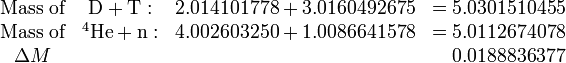$\begin{matrix} \hbox{Mass of}& \mathrm{D} + \mathrm{T}: & 2.014101778+3.0160492675 &= 5.0301510455 \\ \hbox{Mass of}& ^4\mathrm{He}+\mathrm{n}:& 4.002603250+1.0086641578 &= 5.0112674078 \\ \Delta M &&& \quad 0.0188836377 \end{matrix}$

The left hand side in the reaction equation has 0.01888 u more mass than the right hand side. To get an idea of the order of magnitude, we note that the mass of an electron me is 5.485 799 110× 10−4 u, so that ΔM is equal to 34.42 me, i.e., a little over the mass of 34 electrons. In the energy balance ΔM must appear as energy, noting that 1 u = 931.494013 MeV, we find that the energy that comes free in the reaction is ΔU = 17.59 MeV.

We may contrast this nuclear reaction to a typical chemical reaction,

H + H → H2 + 4.5 eV

The left hand side (two free hydrogen atoms) has 4.5 eV more relativistic mass than the hydrogen molecule. The reaction energy 4.5 eV corresponds to 8.8× 10−6 me, which is a completely unobservable loss of mass. The fact that the mass of a molecule is less than the sum of masses of its constituting atoms is true, but such a small effect that it is never included, in, for instance, the translational or rotational energy of the molecule, where the molecular mass plays a role.

## Energy in quantum mechanics

The energy of many (but not all) quantum mechanical systems is quantized, meaning that the energy of the system can take on only discrete values. The historic example of a quantum mechanical system with quantized energies is the one-dimensional harmonic oscillator. The energies are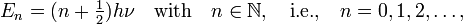$E_n = (n+\tfrac{1}{2}) h \nu\quad \hbox{with}\quad n\in \mathbb{N},\quad\hbox{i.e.,}\quad n=0,1,2,\ldots,$

where h is Planck's constant and ν is the fundamental frequency of the harmonic oscillator; h ν has the dimension energy. According to quantum mechanics it is impossible for the harmonic oscillator to have an energy equal to, e.g., 1.35 h ν, because there is no integer n such that n + ½ = 1.35. Max Planck was forced to introduce this quantized energy expression in his study of black body radiation, in which he assumed the walls of the black body to consist of thermally excited harmonic oscillators. This was the beginning of quantum theory.

As stated, not all energies are quantized, those of unbound systems (scattering systems) are not quantized. A well-known example is the ionization of the hydrogen atom, i.e., the removing of the electron of the H-atom. Once the electron has obtained an energy larger than the ionization potential (13.6 eV), it is a free electron—with a trajectory disturbed by the field of the H-nucleus—that can have any (non-quantized) energy.

In modern quantum mechanics, energy, as any observable physical quantity, is represented by a self-adjoint operator, usually designated by H in honor of William Rowan Hamilton. Most terms in the operator H are obtained from the corresponding classical Hamiltonian (the classical energy expressed in momenta and positions of the particles constituting the system). The momenta are replaced by gradients (times$-i\hbar$ with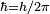$\hbar = h/2\pi$) and the components of the position vectors are simply reinterpreted as multiplicative operators.

### Example

Consider two charged particles of mass m1 and m2, with position vectors r1 and r2, and charges q1 and q2. Classically the particles have kinetic energy and as potential energy the Coulomb (electrostatic) energy:$E_\textrm{clas} = \frac{m_1}{2}\frac{d \mathbf{r}_1}{dt}\cdot\frac{d \mathbf{r}_1}{dt} + \frac{m_2}{2}\frac{d \mathbf{r}_2}{dt}\cdot\frac{d \mathbf{r}_2}{dt} + \frac{q_1\,q_2}{|\mathbf{r}_1-\mathbf{r}_2|}$

This is converted into Hamilton form by defining the momenta of the particles$\mathbf{p}_1 \equiv m_1 \frac{d \mathbf{r}_1}{dt}, \qquad \mathbf{p}_2 \equiv m_2 \frac{d \mathbf{r}_2}{dt},$

and writing the dot products as squares,$\mathbf{p}_1 \cdot \mathbf{p}_1 \equiv p_1^2 \quad \textrm{and} \quad\mathbf{p}_2 \cdot \mathbf{p}_2 \equiv p_2^2$

so that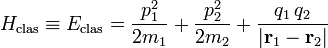$H_\textrm{clas}\equiv E_\textrm{clas} = \frac{ p_1^2}{2m_1} + \frac{p_2^2}{2m_2}+ \frac{q_1\,q_2}{|\mathbf{r}_1-\mathbf{r}_2|}$

Quantization means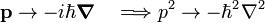$\mathbf{p} \rightarrow -i\hbar\boldsymbol{\nabla} \quad \Longrightarrow p^2 \rightarrow -\hbar^2 \nabla^2$

and reinterpretation of r1 and r2 as multiplicative operators. Here is the gradient, a vector operator known from vector analysis. Hence the quantum mechanical energy operator (Hamilton operator) becomes finally the self-adjoint operator,$H_\textrm{QM} = -\frac{\hbar^2\,\nabla_1^2}{2m_1} - \frac{\hbar^2\,\nabla_2^2}{2m_2}+ \frac{q_1\,q_2}{|\mathbf{r}_1-\mathbf{r}_2|}.$

(The proof that this operator is self-adjoint is omitted). The eigenvalues of this operator are the quantum mechanical energies of this system consisting of two charged particles. The lower energies (below the ionization threshold) are quantized (discrete), those above the ionization threshold are continuous.

Sometimes it is a matter of concern that operators do not commute, while the corresponding classical quantities always commute. Often one can then fall back on the Beltrami form of the Laplace operator for the kinetic energy. Further there are quantum mechanical energy terms that do not have classical counterparts. Commonly these terms depend on electron or nuclear spin. Spin terms can either be derived ad hoc (as in the Wolfgang Pauli's theory of electron spin), or more rigorously by Paul Dirac's relativistic theory.

As was already discussed in the example, the energies En of a quantum mechanical system appear as eigenvalues of the eigenvalue equation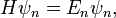$H\psi_n = E_n \psi_n, \,$

which is the time-independent Schrödinger equation. By using n to label the eigenstates ψn, we may suggest the eigenvalues to be discrete, i.e., suggest that n is integral. However, this is not necessary, n may be a continuous label. In that case ψn is usually not normalizable and is referred to as a scattering state.

In quantum mechanical studies the eigenvalue problem of any observable may appear occasionally. However, the observable H (energy) plays a very special—and central—role. Namely, it appears in the fundamental equation of quantum mechanics, Schrödinger's time-dependent equation,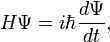$H \Psi = i \hbar \frac{d\Psi}{dt},$

which describes the time evolution of the state function Ψ. This equation is the quantum mechanical counterpart of Newton's second law in classical mechanics and Maxwell's equations in electrodynamics.